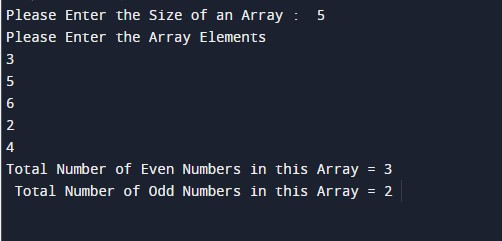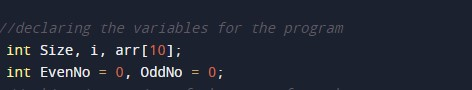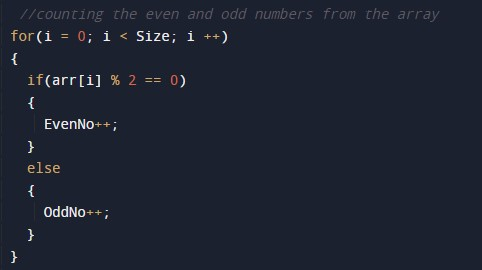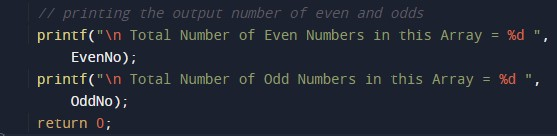C Program to Count Even Odd

In this tutorial you will learn about the C Program to Count Even Odd and its application with practical example.

C Program to Count Even Odd

In this tutorial, we will learn to create a C program that will Count Even Odd in Array using C programming.

Prerequisites

Before starting with this tutorial we assume that you are best aware of the following C programming topics:

• Operators in C Programming.
• Basic Input and Output function in C Programming.
• Basic C programming.
• For loop in C programming.
• Conditional Statements in C programming.
• Arithmetic operations in C Programming.

Program to Count Even Odd in Array

In c programming, it is possible to take numerical input from the user and Count Even Odd with the help of a very small amount of code. The C language has many types of header libraries which has supported function in them with the help of these files the programming is easy.

The Count Even-Odd, Even numbers are those numbers that are completely divisible by 2. which means the reminder should be zero. And odd numbers are those numbers that cannot be completely divisible by 2.

With the help of this program, we can Count Even Odd in Array.

Output:-In the above program, we have first initialized the required variable.• arr= it will hold the integer value.
• i = it will hold the integer value.
• Size= it will hold the integer value.
• EvenNo = it will hold the integer value.
• OddNo= it will hold the integer value.

Input size and elements of the array.Program Logic Code.Printing output Count Even Odd in Array.In this tutorial we have learn about the C Program to Count Even Odd and its application with practical example. I hope you will like this tutorial.